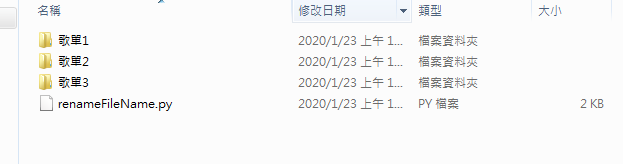#3

## python批量讀取資料夾檔案、修改檔案

python中有好用的`os`模組，

# 使用教學

## 匯入os模組

``````import os
``````

## 一、批量修改檔名

``````# 函數功能: 在指定path路徑下，將該層檔案及資料夾名稱前加上prefix字串
def batch_rename(path, prefix):
for fname in os.listdir(path):
new_fname = prefix+fname
os.rename(os.path.join(path, fname), os.path.join(path, new_fname))
``````

## 二、批量顯示檔名

``````# 函數功能: 顯示指定路徑下，該層的檔案及資料夾名稱
def batch_showname(path):
for fname in os.listdir(path):
print(os.path.join(path, fname))
``````

## 三、遞迴顯示所有檔名

``````# 函數功能: 遞迴顯示指定路徑下的所有檔案及資料夾名稱
def find_dir(path):
for fd in os.listdir(path):
full_path=os.path.join(path,fd)
if os.path.isdir(full_path):
print('資料夾:',full_path)
find_dir(full_path)
else:
print('檔案:',full_path)
``````

# 使用範例``````import os
def find_dir(path):
# 函數功能: 遞迴顯示指定路徑下的所有檔案及資料夾名稱
for fd in os.listdir(path):
full_path=os.path.join(path,fd)
if os.path.isdir(full_path):
print('資料夾:',full_path)
find_dir(full_path)
else:
print('檔案:',full_path)

path="./" #指向當前資料夾的路徑
find_dir(path)
``````

(方便之後將檔案放在同一個資料夾做排序)

``````import os
def batch_rename(path, prefix):
# 函數功能: 在指定path路徑下，將該層檔案及資料夾名稱前加上prefix字串
for fname in os.listdir(path):
new_fname = prefix+fname
os.rename(os.path.join(path, fname), os.path.join(path, new_fname))

batch_rename("./歌單1",'a')
batch_rename("./歌單2",'b')
batch_rename("./歌單3",'c')
``````

# 附上完整程式碼供參考

``````import os
def batch_rename(path, prefix):
# 函數功能: 在指定path路徑下，將該層檔案及資料夾名稱前加上prefix字串
for fname in os.listdir(path):
new_fname = prefix+fname
os.rename(os.path.join(path, fname), os.path.join(path, new_fname))

def batch_showname(path):
# 函數功能: 顯示指定路徑下，該層的檔案及資料夾名稱
for fname in os.listdir(path):
print(os.path.join(path, fname))

def find_dir(path):
# 函數功能: 遞迴顯示指定路徑下的所有檔案及資料夾名稱
for fd in os.listdir(path):
full_path=os.path.join(path,fd)
if os.path.isdir(full_path):
print('資料夾:',full_path)
find_dir(full_path)
else:
print('檔案:',full_path)

path="./" #指向當前資料夾的路徑
find_dir(path)
``````